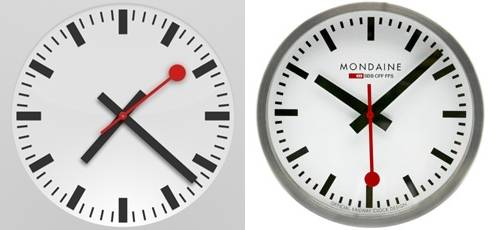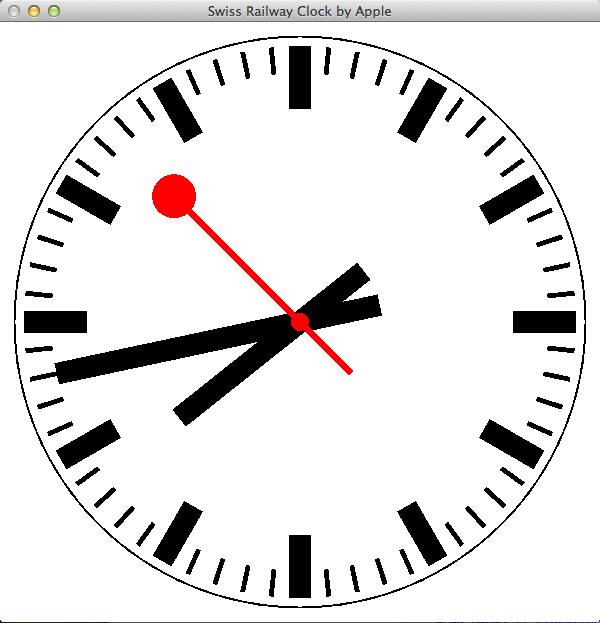# Swiss Railway Clock in HaskellI wanted a small Haskell programming task to solve. I had just read about the controversy around the new Clock application in iOS 6 which is trademarked by the Swiss Federal Railway (see Swiss Railway Clock (Wikipedia)). The clock design is simple and elegant and I though that this would be a nice task to re-implement in Haskell.

I have used gloss for some projects before so this was my first choice to output some graphics. The project was small enough (58 LOC) to be completed in a few evenings.

• I started by measuring the different clock elements from a screenshot of the iOS application.
• Then I defined new types for the clocks hands and time marks which comprised the different characteristics.
• It turns out that most elements are just simple radial lines (`RLine`) through the center of the clock. `draw_line` handles all drawing except the dots on the second hand.
• Gloss support simple animations where all you need to do is to provide is a simple function which produce a `Picture` at a certain time (in seconds) after the program was started. See Graphics.Gloss.Interface.IO.Animate.
• So I get the initial system clock and time zone and feed that into my clock graphics generation function.

This is the resulting code.

``````module Main (main) where
import Graphics.Gloss
import System.Time

type Len = Float
type Angle = Float
type SubSecond = Float
data Face = Face { r_face :: Len, thick :: Len }        -- Center is assumed at origo
data RLine = RLine { len :: Len, width :: Len, r_tip :: Len, col :: Color }
newtype Mark = MarkC { mRLine :: RLine }
newtype Hand = HandC { hRLine :: RLine }
data SecHand = SecHandC { hand :: RLine, r_cent :: Len, r_outer :: Len }

-- Draw RLine at a certain angle. These are drawn through the center of the faceto r_tip.
-- If len > r_tip then the line passes through origo. Angle 0 is represented at 12 a clock direction.
draw_line :: RLine -> Angle -> Picture
draw_line (RLine len width r_tip col) a = Color col \$ Rotate a \$ Translate 0 (-len/2+r_tip) \$
rectangleSolid width len where

-- Draw clock face with a surrounding circle at radius, r and hour and minute marks
draw_face :: Face -> Mark -> Mark -> Picture
draw_face (Face r w) (MarkC h) (MarkC m) = pictures \$ face ++ hour_marks ++ min_marks where
hour_marks = map (\a -> draw_line h a) [0,30..330]
min_marks = map (\a -> draw_line m a) [0,6..354]    -- TODO: Rem. hour marks
face = [ThickCircle r w]

-- Draw clock hands according to calendar time, t
draw_hands :: ClockTime -> SubSecond -> Hand -> Hand -> SecHand -> Picture
draw_hands ct ss (HandC h) (HandC m) (SecHandC s r1 r2) = Color (col s) \$
pictures \$ hour ++ min ++ sec ++ dots where
t = toUTCTime ct
seconds = ss + (fromIntegral \$ ctSec t)     -- set ss=0 if you want distinct seconds
minutes = fromIntegral \$ ctMin t
hours = fromIntegral \$ ctHour t
hour = [draw_line h (30 * (hours + minutes/60))]
min  = [draw_line m (6  * minutes)]
sec  = [draw_line s (6  * seconds)]
dots = [circleSolid r1, Translate sec_x sec_y (circleSolid r2)]
sec_x = (r_tip s) * cos(sec_radians)
sec_y = (r_tip s) * sin(sec_radians)
sec_radians = (pi/2) - (pi/30) * seconds

-- Get current local time zone diff in hours
get_timezone :: ClockTime -> IO Int
get_timezone ct = do cal <- toCalendarTime ct
return ((ctTZ cal) `div` 3600)

-- Draw clock with clock time (ct) and timezone (tz) (hours) and a time diff (td) (seconds) since start
drawClock :: ClockTime -> Int -> Float -> Picture
drawClock ct tz td = Translate trans trans \$ Scale scale scale \$ picture
where
size = 600
scale = 0.4
trans = -0.0*(fromIntegral size)
picture = pictures [draw_face face m_hour m_min, draw_hands ct' subsec h_hour h_min h_sec]
-- Ugly way to handle timezone. This works because draw_hands use toUTCTime
ct' = addToClockTime (TimeDiff	0 0 0 tz 0 sec 0) ct
(sec, subsec) = properFraction td
face = Face 714 5
m_hour = MarkC \$ RLine 158 54 690 black
m_min = MarkC \$ RLine 68 12 690 black
h_hour = HandC \$ RLine 590 54 386 black
h_min = HandC \$ RLine 826 54 622 black
h_sec = SecHandC (RLine 626 16 445 red) 23 55

main = do
ct <- getClockTime
tz <- get_timezone ct
animate
(InWindow "Swiss Railway Clock by Apple" (600,600) (100,100))
white                   -- background color
(drawClock ct tz)       -- picture to display
``````

A screenshot of the resulting clock shown below. Unfortunately gloss does not yet support anti-aliasing which would make the graphics more smooth.I have generated a binary application for MacOS Lion.

To generate a new binary i used the following commands.

``````ghc -O2 -dylib-install-name /Applications/Xcode.app/Contents/Developer//Platforms/MacOSX.platform/Developer/SDKs/MacOSX10.7.sdk/usr/lib AppleClock.hs
strip AppleClock -o AppleClockStrip

./AppleClockStrip
``````